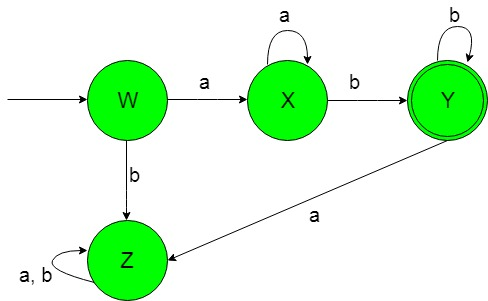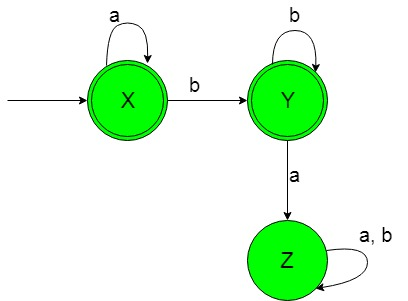# Designing Deterministic Finite Automata (Set 6)

Prerequisite: Designing finite automata
In this article, we will see some designing of Deterministic Finite Automata (DFA).

Problem-1: Construction of a minimal DFA accepting set of strings over {a, b} in which anbm, where n and m is greater than or equal to 1.
Explanation: The desired language will be like:

`L1 = {ab, aab, abb, aabb, aaabbb, aaabbbb, ...........}`

Note: In the above string there should not be any ‘a’ after ‘b’.

Here as we can see that each string of the language containing a and b whose power is greater or equal to 1 but the below language is not accepted by this DFA because some of the string of the below language does not containing a and b whose power is greater or equal to 1.

`L2 = {ε, a, b, ..............}`

This language L2 is not accepted by this required DFA.
The state transition diagram of the desired language will be like below:In the above DFA, the state ‘W’ is the initial state and which on getting ‘a’ as the input it transit to the normal state ‘X’ which on getting ‘a’ as the input it remains in the state of itself and on getting ‘b’ as the input it transit to the final state ‘Y’ which on getting ‘b’ as the input it remains in the state of itself and on getting as the input it transit to the dead state ‘Z’ and when initial state ‘W’ gets ‘b’ as the input then it transit to the dead state ‘Z’.

The state ‘Z’ is called dead state because it can not go to the final state on getting any input.

Problem-2: Construction of a minimal DFA accepting set of strings over {a, b} in which anbm, where n and m is greater than or equal to 0.
Explanation: The desired language will be like:

`L1 = {ε, a, b, ab, aab, abb, aabb, aaabbb, aaabbbb, ...........}`

Note: In the above string there should not be any ‘a’ after ‘b’.

Here as we can see that each string of the language containing a and b whose power is greater or equal to 0 but the below language is not accepted by this DFA because some of the string of the below language does not contain a and b whose power is greater or equal to 0 or they might not follow the format of a and b i.e, there should not be any ‘a’ after ‘b’.

`L2 = {ba, baa, bbaaa..............}`

This language L2 is not accepted by this required DFA because it’s string contain ‘a’ after ‘b’.
The state transition diagram of the desired language will be like below:In the above DFA, the state ‘X’ is the initial and final state which on getting ‘a’ as the input it remains in the state of itself and on getting ‘b’ as the input it transit to the final ‘Y’ which on getting ‘b’ as the input it remains in the state of itself and on getting ‘a’ as the input it transit to dead state ‘Z’.

The state ‘Z’ is called a dead state because it can not go to the final state on getting any input alphabet.